Open In App

# Probability in Maths

Probability in Maths is mainly concerned with the possibility of events. In other words, probability tells us about the likelihood of events. The probability value always falls between 0 and 1. It tells us whether an event will happen or not. When we cannot tell whether a particular thing will happen or not, we use probability.

In this article, you will learn about Probability, its definition, Formula, Terminology, Types of Probability, Bayes’ Theorem, and other things.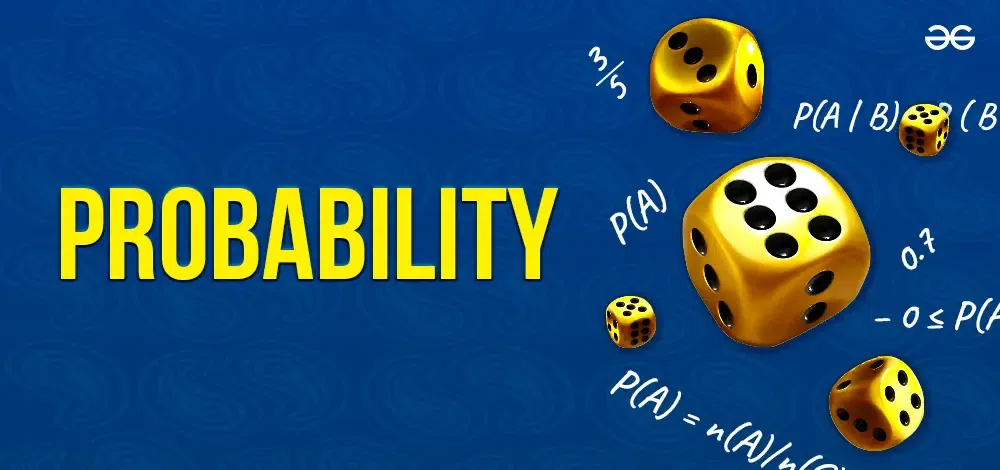Table of Content

## Definition of Probability in Mathematics

Probability is a way to analyze how probable something is to happen. Many occurrences are difficult to predict with 100 percent accuracy. We can only estimate the possibility of an event occurring. The values range from 0 to 1, with 0 telling us improbability and 1 denoting certainty. For calculating probability, we simply divide the number of favorable outcomes to the total number of outcomes.

## Terminology of Probability Theory

### 1. Sample Space

The set of every possible outcome in a probability experiment(trail)is known as the sample space. For example, the sample space for tossing a coin is head and tail.

### 2. Sample Point

It is one of the possible results. Consider the example of rolling a fair six-sided dice. The sample space for this experiment consists of six possible outcomes: 1,2,3,4,5 or 6. Each of these possible outcomes is a sample point.

### 3. Experiment

A process or trial that provides a range of potential results is called an experiment. Any scenario where the results are uncertain may be the subject of the experiment. For example, The tossing of a coin, Selecting a card from a deck of cards, throwing a die, etc.

### 4. Event

A subset of the sample space. For example: getting 1 as an outcome while rolling a die is an event.

### 5. Favorable outcome

An occurrence that has produced the desired consequence or an expected event.

### 6. Equally Likely Events

Equally likely events are those whose chances or probabilities of happening are equal. Both events are not related to one another. For example, there are equal possibilities of receiving either a head or a tail when we flip a coin.

### 7. Exhaustive Events

We call an event exhaustive when the set of all experiment results is the same as the sample space.

### 8. Mutually Exclusive Events

Events that are mutually exclusive cannot occur at the same time. For instance, the weather may be hot or chilly simultaneously. We can’t have the same weather at the same time.

### 9. Complimentary events

The non-occurring events. The complement of an event A is the event, not A(or A’).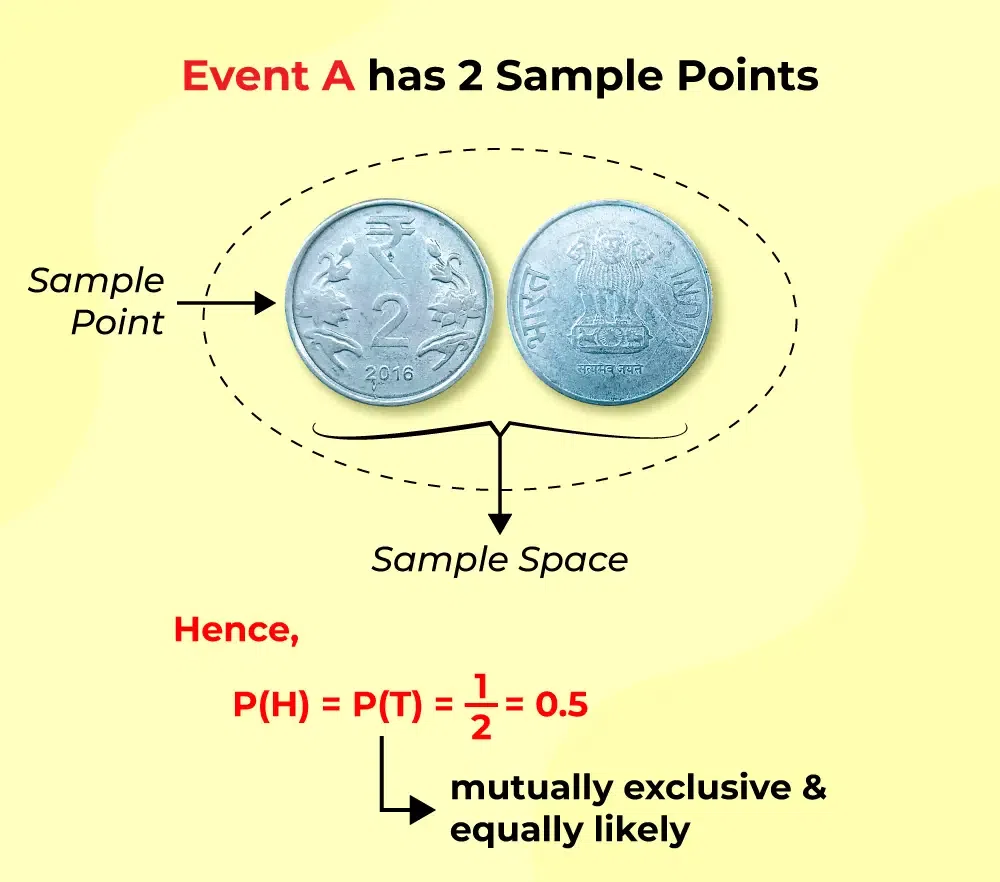## Probability Density Function

We define the Probability Distribution function as a function that contains all the possible outcomes of any given situation. It is also known as the Probability Distribution Function

## Probability Formula

The formula defines the possibility that an event will occur. It is defined as the ratio of the number of favorable outcomes to the total number of outcomes.

The probability formula can be expressed as:

P(E) = Number of favorable outcomes / Total number of outcomes

where P(E) denotes the probability of an event E.

### Solved Examples

Example 1. There are 8 balls in a container, 4 are red, 1 is yellow and 3 are blue. What is the probability of picking a yellow ball?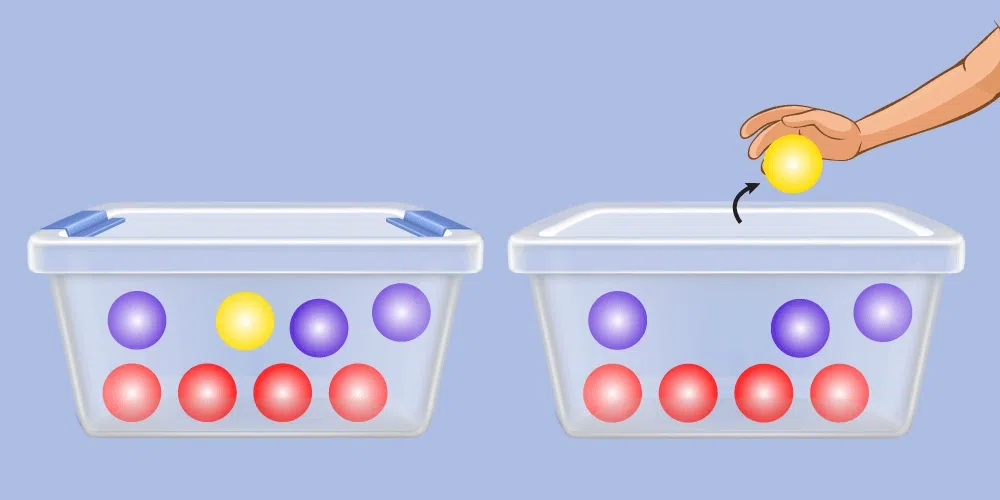The probability is equal to the number of yellow balls in the container divided by the total number of balls in the container, i.e. 1/8.

Example 2: A dice is rolled. What is the probability that an even number has been obtained?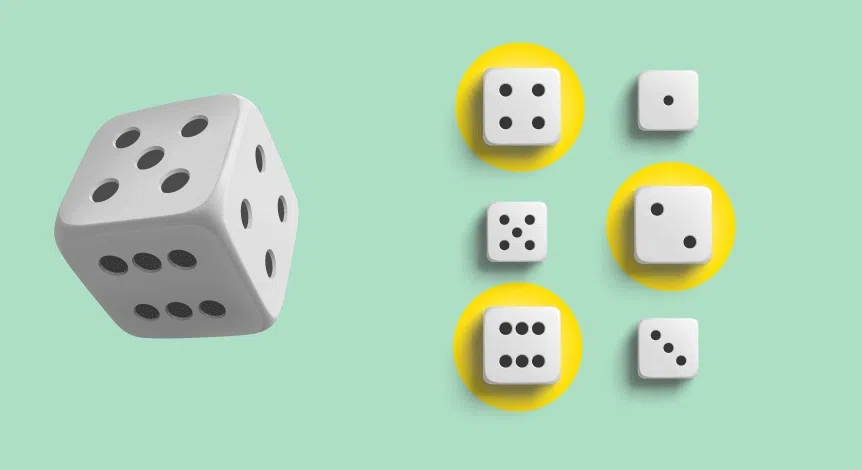When fair six-sided dice are rolled, there are six possible outcomes: 1, 2, 3, 4, 5, or 6.

Out of these, half are even (2, 4, 6) and half are odd (1, 3, 5). Therefore, the probability of getting an even number is:

P(even) = number of even outcomes / total number of outcomes

P(even) = 3 / 6

P(even) = 1/2

### Probability Tree Diagram

A tree diagram in probability is a graphic representation that helps us in determining the likely outcomes that is whether an event will occur or not. It helps us understand the all possibilities of an event and which possibilities can occur and cannot occur.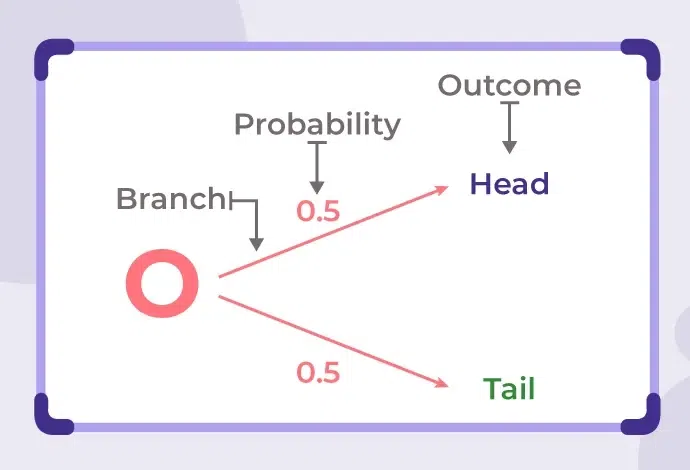## Types of Probability

There are 3 major distinctions in which probability can be differentiated:

1. Theoretical Probability
2. Experimental Probability
3. Axiomatic Probability

### Theoretical Probability

It is focused on the likelihood of anything occurring. The reasoning behind probability is the foundation of scientific probability. If a coin is flipped, the statistical chance of having a head is 1/2. An event’s statistical chance is the chance that the occurrence will occur. It is determined by dividing the number of desirable outcomes by the total number of outcomes is thus termed theoretical probability and can be learned in the following articles:

### Experimental Probability

It is founded on the results of an experiment. The experimental chance can be determined by dividing the total number of trials by the number of potential outcomes. For example, if a coin is flipped ten times and heads are reported six times, the experimental chance of heads is 6/10 or 3/5. Let’s discuss the experimental probability in the following articles:

### Axiomatic Probability

A collection of laws or axioms that apply to all forms is established in axiomatic probability. Kolmogorov developed these axioms, which are known as Kolmogorov’s three axioms. The axiomatic approach to probability quantifies the likelihood of events occurring or not occurring. This definition is covered in detail in the axiomatic probability articles, which include Kolmogorov’s three laws (axioms) as well as numerous examples. The chance of an event or result happening depending on the existence of a prior event or outcome is known as conditional probability. Following are the articles based on the axiomatic probability:

## Probability Theorems

The following theorems of probability are helpful to understand the applications of probability and also perform the numerous calculations involving probability.

Theorem 1: The sum of the probability of happening an event and not happening an event is equal to 1.                                                                                      P(A) + P(A’) = 1

Theorem 2: The probability of an impossible event or the probability of an event not happening is always equal to 0.

P(ϕ) = 0

Theorem 3: The probability of a sure event is always equal to 1.

P(A) = 1

Theorem 4: The probability of happening of any event always lies between 0 and 1.

0 < P(A) < 1

Theorem 5: If there are two events A and B, we can apply the formula of the union of two sets and we can derive the formula for the probability of happening of event A or event B as follows.

P(A∪B) = P(A) + P(B) − P(A∩B)

Also for two mutually exclusive events A and B, we have P( A U B) = P(A) + P(B)

We have a few more formulas used to calculate the probability of any event :

1. Whenever an event is the union of two  events, say A and B, then
P(A or B) = P(A) + P(B) – P(A∩B)
P(A ∪ B) = P(A) + P(B) – P(A∩B)
2.  Whenever an event is the complement of another event, if A is an event, then P(not A) = 1 – P(A) or P(A’) = 1 – P(A).
P(A) + P(A′) = 1.
3. When event A has already taken place and the probability of event B is to be calculated, then P(B, given A) = P(A and B), P(A, given B). It can be vice versa in the case of event B.
P(B∣A) = P(A∩B) / P(A)
4.  Whenever an event is the intersection of two events, that is, events A and B need to occur simultaneously. Then P(A and B) = P(A)⋅P(B).
P(A∩B) = P(A)⋅P(B∣A)

## Bayes’ Theorem on Conditional Probability

Bayes’ Theorem defines the probability of an event based on the condition of occurrence of other events. It is also called conditional probability. It helps in calculating the probability of occurrence of one event based on the condition that another event is also taking place or occurring. It is named after Thomas Bayes who was an English statistician and is used widely in machine learning, mathematics, and statistics.

For example, let us assume you have two bags of marbles in various colors. Bag A has three red and two blue marbles, while bag B has one red and four blue marbles. You pick one of the bags at random, then you pick a marble at random from that bag. Given that you selected a red marble, what is the probability that you selected bag A? Such probability is called conditional probability.

The formula for Bayes’ Theorem is :

P(A|B) = P(B|A)⋅P(A) P(B)

where P(A|B) denotes how often event A happens on a condition that B happens.

where P(B|A) denotes how often event B happens on a condition that A happens.

P(A) is the probability of event A occurring.

P(B) is the probability of event B occur

## Coin Toss Probability

Now let us take into account the case of coin tossing to understand probability in a better way.

### Tossing a Coin

A single coin when flipped has two possible outcomes, a head or a tail. The definition of probability when applied here to find the probability of getting a head or getting a tail.

The total number of possible outcomes = 2

Sample Space = {H, T} H: Head, T: Tail

• P(H) = Number of Heads/ Total Number of outcomes = 1/2
• P(T) = Number of Tails/ Total Number of outcomes = 1/2

### Tossing Two Coins

There are a total of four possible results when tossing two coins. We can calculate the probability of two heads or two tails using the formula.

The probability of getting two tails can be calculated as :

Total number of outcomes = 4

Sample Space = {(H,H),(H,T),(T,H),(T,T)}

• P(2T) = P(0 H) = Number of outcomes with two tails/ Total number of outcomes = 1/4
• P(1H) = P(1T) = Number of outcomes with one head/ Total number of outcomes = 2/4 = 1/2

### Tossing Three Coins

The number of total outcomes on tossing three coins is 23 = 8. For these outcomes, we can find the probability of various cases such as getting one tail, two tails, three tails, and no tails, and similarly can be calculated for several heads.

Total number of outcomes = 23 = 8

Sample space = {(H, H, H), (H, H, T),(H, T, H), (T, H, H), (T, T, H), (T, H, T), (H, T, T), (T, T, T)}.

P(3T) = P(0 H) = Number of outcomes with three tails/ Total Number of outcomes = 1/8

### Dice Roll Probability

Various games use dice to decide the movements of the player during the games. A dice has six outcomes. Some games are played using two dice. Now let us calculate the outcomes, and their probabilities for one dice and two dice respectively.

### Rolling One Dice

The number of outcomes is 6 when a die is rolled and the sample space is = {1,2,3,4,5,6}.

Let us now discuss some cases

• P(Even Number) = Number of outcomes in which even number occur/Total Outcomes = 3/6 = 1/2
• P(Prime Number) = Number of prime number outcomes/ Total Outcomes = 3/6 = 1/2

### Rolling Two Dice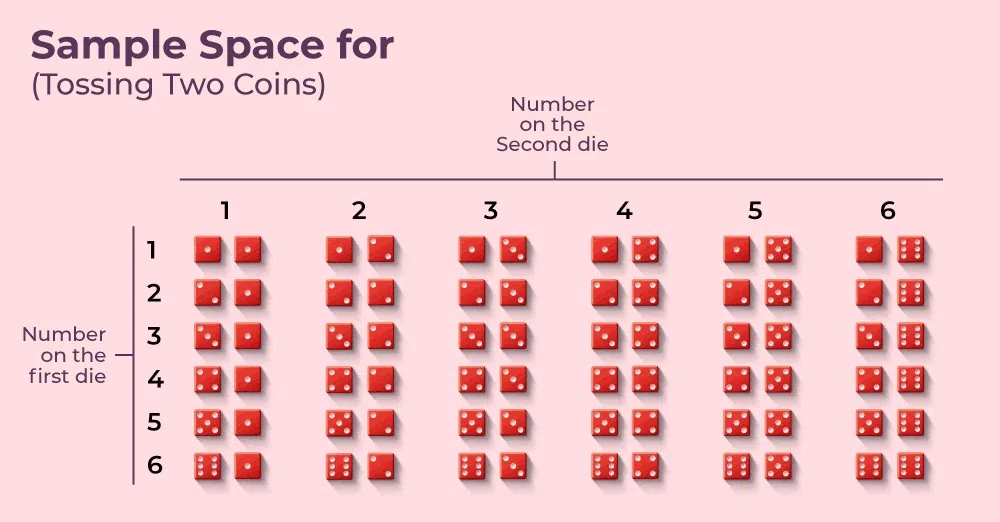The number of total outcomes, when two dice are rolled, is 62 = 36.

Let us check a few cases in the above example,

• Probability of getting a doublet(Same number) = 6/36 = 1/6
• Probability of getting a number 3 on at least one dice = 11/36

### Probability of Drawing Cards

Spades, clubs, diamonds, and hearts make up the four suits that form a deck of 52 playing cards. There are a total of 52 cards, with 13 in each of the four suits (clubs, diamonds, hearts, and spades). The symbols for the cards are listed below.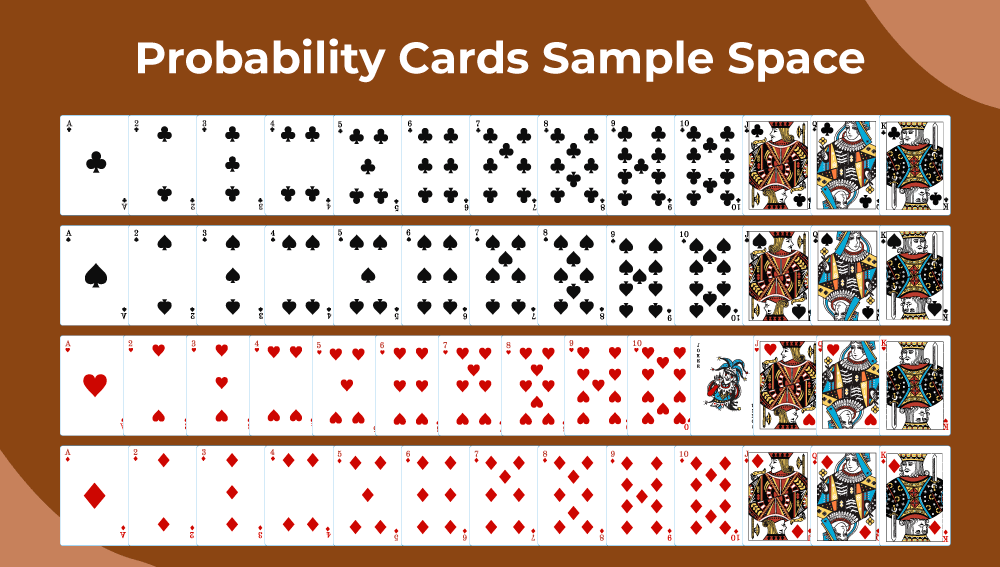Black cards are spades and clubs. Red cards are hearts and diamonds. Ace, 2, 3, 4, 5, 6, 7, 8, 9, 10, Jack, queen, and King are the 13 cards that make up each suit. The jack, queen, and king are referred to as face cards in these.

• Probability of getting a black card is P(Black Card) = 26/52 = 1/2
• Probability of getting a heart is P(Heart) = 13/52 = 1/4
• Probability of getting a face card is P(Face card) = 12/52 = 3/13

## Sample Problems on Probability

Problem 1. Tickets numbered 1 to 20 are mixed up and then a ticket is drawn at random. What is the probability that the ticket drawn has a number which is a multiple of 3 or 5?

Solution:

Here S is {1,2,3,4,5,6,7,8………19,20}.

Favorable outcomes(E) = {3,5,6,9,10,12,15,18,20}

P(getting multiple of 3 or 5) = n(E)/n(S) = 9/20.

Problem 2. In a lottery, there are 10 prizes and 25 blanks. A lottery is drawn at random. What is the probability that a blank is selected?

Solution:

S= Total outcomes

E= getting a blank.

n(E) = 25

P(Getting a blank) = n(E)/n(S) = 25/35 = 5/7.

Problem 3. A bag contains 4 white, 5 red, and 6 blue balls. Three balls are drawn at random from the bag. The probability that all of them are red, is:

Solution:

Let S be the sample space.

Then, n(S) = Number of ways of drawing 3 balls out of 15

= 15C3 = 455

Let E = event of getting all the 3 red balls.

n(E) = 5C3 = 10

P(E) = n(E)/n(S) = 10/455 = 2/91.

Problem 4. In a class there are 10 girls and 15 boys, what is the probability that 1 girl and 2 boys are selected?

Solution:

Let S be the sample space.

Then, n(S)= Number of ways of selecting 3 children out of 25

= 25C3

= 2300.

Let  E= event of selecting 1 girl and 2 boys.

n(E) = 10C1*15C2 = 1050

P(E) = n(E)/n(S) = 1050/2300 = 21/46.

## FAQs on Probability in Maths

### Q1. What is Probability? Give an example.

Probability is a branch of mathematics that is a measure of how probable an incident is to occur. For example, when a coin is tossed in the air, there are two possible outcomes head or tail.

### Q2. How numerous types of Probability are there?

There are three types of probability :

1. Theoretical Probability
2. Experimental Probability
3. Axiomatic Probability

### Q3. What is the formula for Probability?

P(E) = Number of favorable outcomes /Total number of outcomes

where P(E) denotes the probability of an event E.

### Q4. What does the Probability of 0 means?

The probability of zero denotes the event does not take place.